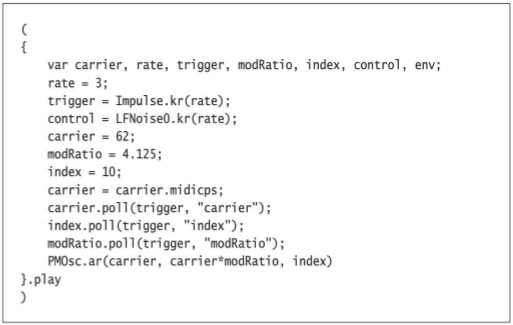# Examples from supercollider book

ANy reason why ceratin exampels give an error``````(
{
var carrier, rate, trigger, modRatio, index, control, env;
rate= 3;
trigger=Impulse.kr(rate);
control+LFNoise0.kr(rate);
carrier= 62;
modRatio=4.125;
index=10;
carrier=carrier.midicps;
carrier.poll(trigger, "carrier");
index.poll(trigger,  "index");
modRatio.poll(trigger, "modRatio");
PMOsc.ar(carrier, carrier*modRatio, index)
}.play
)``````

The error message exactly shows the reason:

ERROR: Message ‘+’ not understood.
nil
ARGS:
Instance of LFNoise0 { (0x11b28f978, gc=6C, fmt=00, flg=00, set=03)
instance variables 
synthDef : instance of SynthDef (0x11de65878, size=16, set=4)
inputs : instance of Array (0x123657bf8, size=1, set=2)
rate : Symbol ‘control’
synthIndex : Integer 2
specialIndex : Integer 0
antecedents : nil
descendants : nil
widthFirstAntecedents : nil
}

Error messages are often cryptic, but here it points to the source immediately: `control` equals zero, because it hasn’t been assigned something meaningful.

This is a typo. `control = something` and `control + something` naturally don’t mean the same thing and aren’t interchangeable.

The original example is fine.

hjh